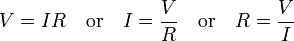# Simple Algebraic Solution.

Gold Member

## Homework Statement

Right now I am at the very beginning of what I am sure will be a very long process of getting my Electrical Engineering Degree. I am currently taking math 1010 online, and it is currently asking this question.

After completing all the tasks required for this module on Systems of Linear Equations, Inequalities, and Problem Solving do the following:

Think of your chosen field (or if you have not yet chosen a major, pick a field you are interested in). Find an application that would involve the algebraic skills learned in this module. Post the application as well as the solution.

You may find the application anywhere you'd like (books, Internet, person in field etc.)

So, I am hoping to ask a person in the field of Electrical Engineering. It seems that most EE problems are much more advanced mathematically than simple Linear Equations.

If anyone can offer their input I would be greatly appreciative.

## Homework Equations

A similar equation is the "A Train left a station doing such and such," but I don't want to use that, as everyone else in the class is doing some variation of it.

## The Attempt at a Solution

I think it's not a good idea to pick a problem from EE problems. Because most of it are boring.

Gold Member
Actually, I think most of them are too difficult for me to comprehend at this early stage...

I ended up creating a function for draining and refilling a swimming pool. (like that isn't boring)

Ok, take this example

Two important things you should know
1-http://en.wikipedia.org/wiki/Ohm%27s_law" [Broken]2-http://en.wikipedia.org/wiki/Kirchhoff%27s_circuit_laws" [Broken]

Using Ohm's and Kirchhoff's Current Laws, you will end up with a system of four equations and four unknowns.

Also,you can modifies the question so that you have complex numbers in the four equations.

Last edited by a moderator: